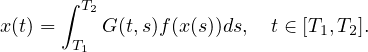Electron. J. Differential Equations, Vol. 2019 (2019), No. 99, pp. 1-20.

### Avery fixed point theorem applied to Hammerstein integral equations Paul W. Eloe, Jeffrey T. Neugebauer

Abstract:
We apply a recent Avery et al. fixed point theorem to the Hammerstein integral equationUnder certain conditions on G, we show the existence of positive and positive symmetric solutions. Examples are given where G is a convolution kernel and where G is a Green's function associated with different boundary-value problem.

Submitted November 28, 2018. Published August 13, 2019.
Math Subject Classifications: 47H10, 34A08, 34B15, 34B27, 45G10.
Key Words: Hammerstein integral equation; boundary-value problem; fractional boundary-value problem.

Show me the PDF file (370 KB), TEX file for this article.Paul W. Eloe Department of Mathematics University of Dayton Dayton, OH 45469, USA email: peloe1@udayton.edu Jeffrey T. Neugebauer Department of Mathematics and Statistics Eastern Kentucky University Richmond, KY 40475, USA email: Jeffrey.Neugebauer@eku.edu